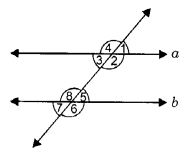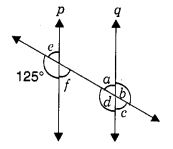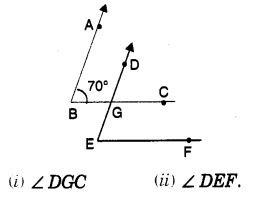NCERT Solutions for Class 7 Maths Chapter 5 Lines and Angles Ex 5.2 are part of NCERT Solutions for Class 7 Maths. Here we have given NCERT Solutions for Class 7 Maths Chapter 5 Lines and Angles Ex 5.2.

 Board CBSE Textbook NCERT Class Class 7 Subject Maths Chapter Chapter 5 Chapter Name Lines and Angles Exercise Ex 5.2 Number of Questions Solved 6 Category NCERT Solutions

## NCERT Solutions for Class 7 Maths Chapter 5 Lines and Angles Ex 5.2

Question 1.
State the property that is used in each of the following statements?

1. If a || b, then ∠ 1 = ∠ 5.
2. If ∠ 4 = ∠ 6, then a || b.
3. If ∠ 4 + ∠ 5 = 180°, then a || b.Solution:

1. Corresponding angle property.
2. Alternate interior angle property.
3. Interior angles on the same side of the transversal are supplementary.

Question 2.
In the following figure, identify:1. the pairs of corresponding angles.
2. the pairs of alternate interior angles.
3. the pairs of interior angles on the same side of the transversal.
4. the vertically opposite angles.Solution:

1. ∠1, ∠5; ∠2, ∠6; ∠3, ∠7 and ∠4, ∠8 are four pairs of corresponding angles.
2. ∠2, ∠8, and ∠3, ∠5 are two pairs of alternate interior angles.
3. ∠2, ∠5, and ∠3, ∠8 are two pairs of interior angles on the same side of the transversal.
4. ∠1, ∠3; ∠2, ∠4; ∠5, ∠7 and ∠6, ∠8 are four pairs of vertically opposite angles.

Question 3.
In the adjoining figure, p || q. Find the unknown angles.
Solution:
a = 55°, b = 125°, c = 55°, d = 125°, e = 55°, f = 55°.

Question 4.
Find the value of x in each of the following figures if l || m.Solution:
(i) Since, l || m and t is a transversal.
∴ ∠x = (180° – 110°) = 70° [Corresponding angles, Linear pair]

(ii) if l || m and a is a transversal.
Then, ∠x = 1000 [Corresponding angles]

Question 5.
In the given figure, the arms of two angles are parallel. If ∠ ABC = 70°, then findSolution:

1. 70°
2. 70°

Question 6.
In the given figures below, decide whether l is parallel to m.Solution:

1. l is not parallel to m
2. l is not parallel to m
3. l || m
4. l is not parallel to m

We hope the NCERT Solutions for Class 7 Maths Chapter 5 Lines and Angles Ex 5.2 help you. If you have any query regarding NCERT Solutions for Class 7 Maths Chapter 5 Lines and Angles Ex 5.2, drop a comment below and we will get back to you at the earliest.